We have seen that the parallel adder circuit built using a cascaded arrangement of full adders is highly affected by the delay associated with the propagation of carry bits (more on this in the article “Parallel Adder”). Greater the number of bits needed to be added, greater is the delay associated. If we need to get rid of this ‘delay’ problem, then we need to go for the design of Look Ahead Carry Adder circuit.

In order to overcome the ripple carry propagation delay, one solution is to anticipate well in advance, about the cases which would lead to the generation of the carry bits. The earlier done, the better it would be. Hence, if we can find whether we get a carry or not just by looking at the input bits which require to be added, it would be the best.

Now suppose that we have the input bits to be added as 0 and 0 or 1 and 0 or 0 and 1, then the sum would be 0 or 1 or 1 and there would be no carry. On the other hand, if both of the input bits are 1, then the sum would be 1 and there would be a carry generated. It is to be noted that these statements are true only if the carry-in (ci) bit which requires to be added with the input bits is zero. Now let us assume that this ci is 1. In this situation, the carry will be generated in all the cases (combination of 0 and 1, 1 and 0 and 1 and 1) except when both of the input bits are 0.
Combining these two factors, it can be concluded that we would get our carry term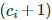under two situations: (i) when both of the input bits (ai, bi) are 1 or (ii) when any one of the input bits (ai, bi) is 1 and the carry obtained by adding the previous input bits (ci) is 1.

These statements can be equivalently represented in terms of logical expressions as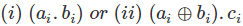Collectively, the expression for the carry bit can be written as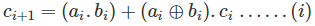However we have our logical expression for the sum kept as such i.e.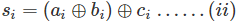Next, let us denote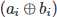by the term Pi called carry-propagation and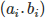by the term Gi called carry-generation. Thus the equations (i) and (ii) can be rewritten as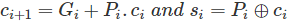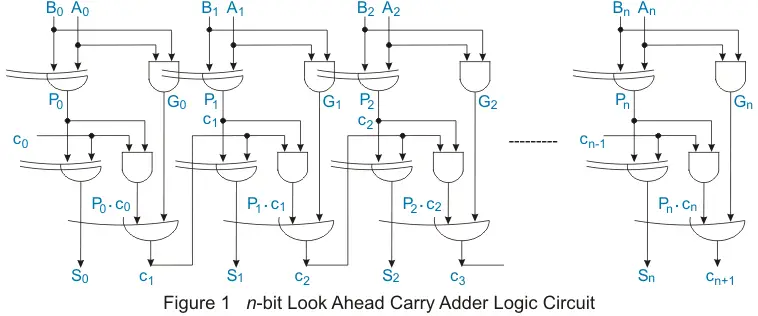Specifically,
For i = 0, we have,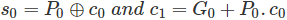For i = 1, we have,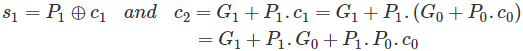For i = 2, we have,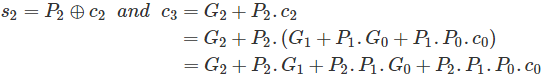Generalizing, for i = n, we have,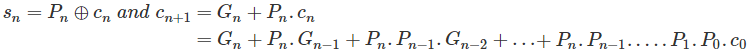The important factor to be noticed in all of the above expressions is the fact that the carry term of any particular stage depends only on the carry-input (c0) term apart from carry-generation and carry-propagation terms. Yes, only on c0 (a user provided input bit) and not the carry terms generated by its preceding stages. This is true irrespective of the stage to which they belong.
This is different from the case of ripple carry adders/subtractors as they have the carry term of their present stage decided by the carry term of their immediate preceding stage. This quality of carry look ahead adder makes it overcome the ripple carry propagation delay associated with normal adder/subtractor circuits.
The same reason enables look ahead adders to operate much faster in comparison to other kinds. However the price paid for it is the complexity involved in its hardware.

Want To Learn Faster? 🎓
Get electrical articles delivered to your inbox every week.
No credit card required—it’s 100% free.